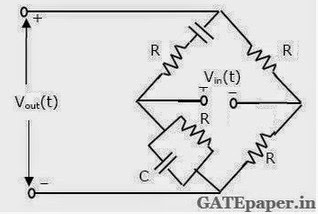### GATE 1994 ECE Analog Circuits (Analog Electronics) - Video Solutions

1. A Ramp voltage v(t) = 100t volts is applied to a RC differentiating circuit with R = 5 kΩ and C = 4 µF. The maximum output voltage is ______
a. 0.2 volts
b. 2.0 volts
c. 10.0 volts
d. 50.0 volts

2. A class-A transformer coupled transistor power amplifier is required to deliver a power output of 10 watts. The maximum power rating of the transistor should not be less than ______
a. 5 watts
b. 10 watts
c. 20 watts
d. 40 watts

3. In order to reduce the harmonic distortion in an amplifier, its dynamic range has to be _________

4. A common emitter transistor amplifier has a collector current of 1.0 mA when its base current is 25 µA at room temperature. Its input resistance is approximately equal to _______

5. A pulse having a rise time of 40 nsec is displayed on a CRO of 12 MHz bandwidth. The rise time of the pulse as observed on the CRO would be approximately equal to ____________

6. For the 2N338 transistor, the manufacturer specifies Pmax = 100 mW at 25oC, free air temperature and the maximum junction temperature Tjmax = 125oC. Its thermal resistance is ________

7. The frequency compensation is used in operational amplifiers is to increase its ___________

8. Match the following:
a) Hartley 1) Low frequency oscillator
b) Wein-bridge 2) High frequency oscillator
c) Crystal 3) stable frequency oscillator
4) Relaxation frequency oscillator
5) Negative resistance oscillator

9. Assuming that the amplifier shown in the figure below is a voltage controlled voltage source.
Show that the voltage transfer function of the network is given by10. Calculate the frequency at which zero transmission is obtained from the Wien-bridge shown below.11. Find the output voltage of the following circuit shown below, assuming ideal operational amplifier behavior.12. In the MOSFET amplifier shown in the figure below, the transistor has µ = 50, rd = 10 kΩ, Cgs = 5 pF, Cgd = 1 pF and Cds = 2 pF.Draw a small signal equivalent circuit for the amplifier for mid band calculate its mid band voltage gain.